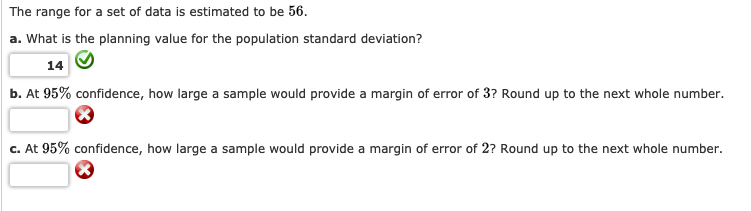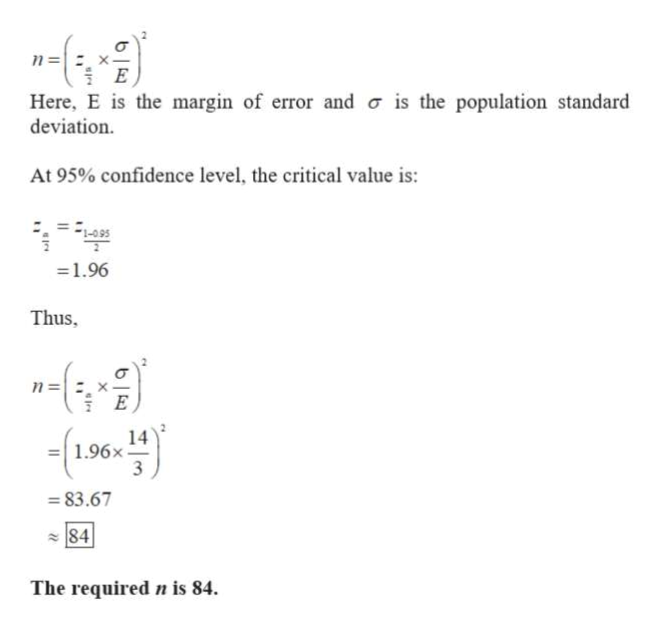# The range for a set of data is estimated to be 56.a. What is the planning value for the population standard deviation?14b. At 95% confidence, how large a sample would provide a margin of error of 3? Round up to the next whole numberc. At 95% confidence, how large a sample would provide a margin of error of 2? Round up to the next whole number.

Questionhelp_outlineImage TranscriptioncloseThe range for a set of data is estimated to be 56. a. What is the planning value for the population standard deviation? 14 b. At 95% confidence, how large a sample would provide a margin of error of 3? Round up to the next whole number c. At 95% confidence, how large a sample would provide a margin of error of 2? Round up to the next whole number. fullscreen
check_circle

Step 1

a. The planning value for the standard deviation is calculated as:

Step 2

b. The required sample size (n) for estimating populati...help_outlineImage Transcriptionclosen= = x- 물 E Here, E is the margin of error and o is the population standard deviation At 95% confidence level, the critical value is: - 1.96 Thus n= E 14 1.96x 3 83.67 84 The required n is 84 fullscreen

### Want to see the full answer?

See Solution

#### Want to see this answer and more?

Solutions are written by subject experts who are available 24/7. Questions are typically answered within 1 hour.*

See Solution
*Response times may vary by subject and question.
Tagged in

### Other